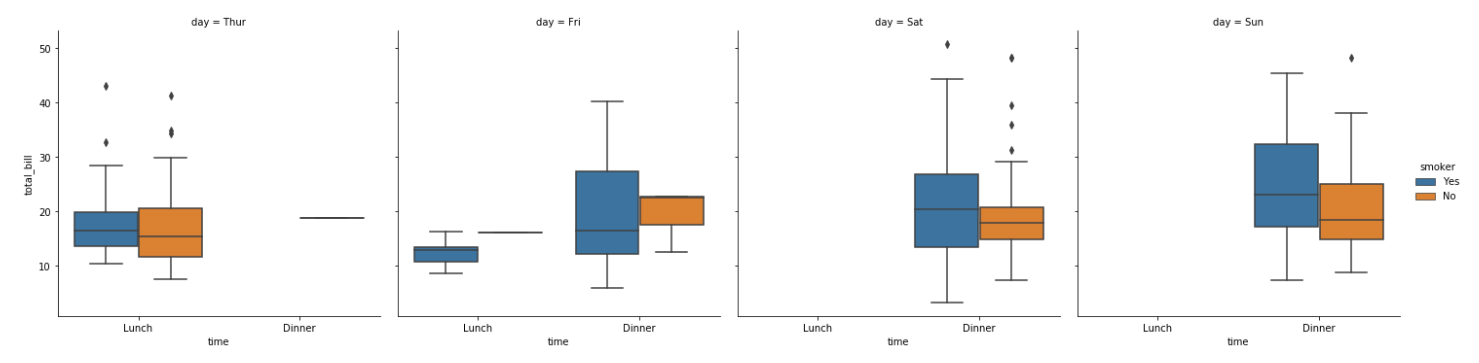# seaborn 高级绘图

``````import numpy as np
import pandas as pd
import matplotlib.pyplot as plt
import seaborn as sns
from scipy import stats,integrate
``````

## 单变量数据

``````x = np.random.normal(size=100)
``````
``````sns.distplot(x, kde=False)
``````
• x：表示所需要绘制的数据集
• kde：是否绘制高斯核密度估计，默认为 True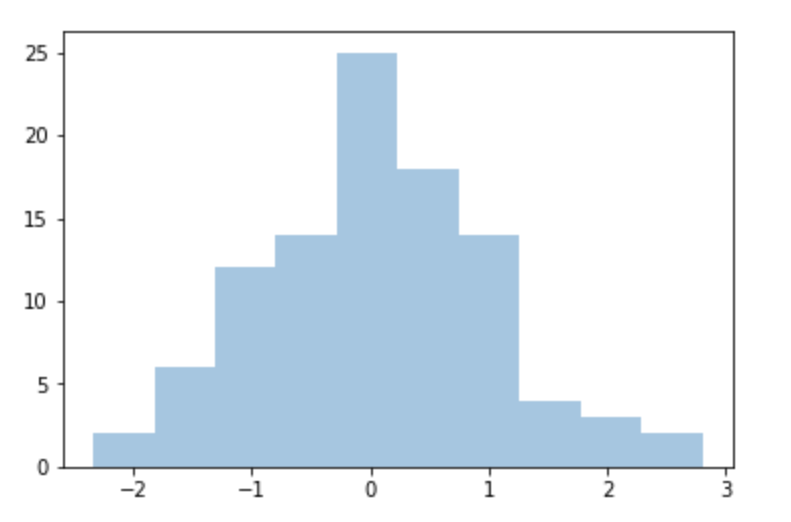``````sns.distplot(x, bins=20)
``````
• x：表示所需要绘制的数据集
• bins：数据显示为多少个柱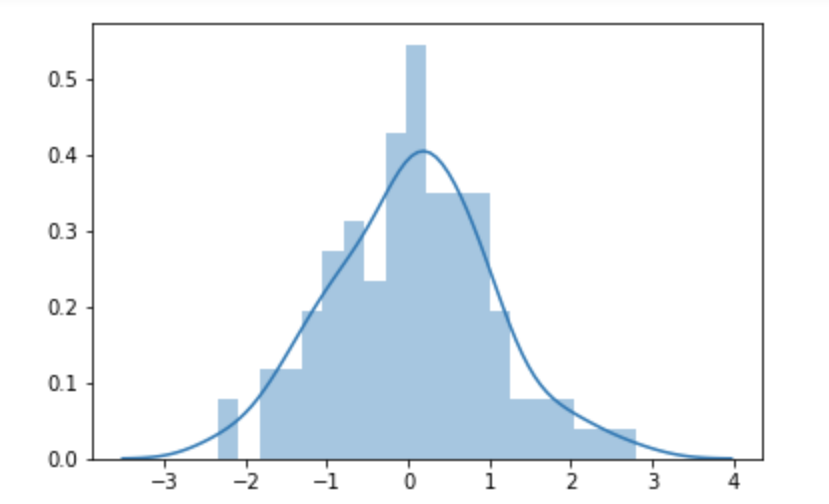``````# 生成 gamma 分布数据
x = np.random.gamma(6, size=200)
# 使用fit 属性来拟合 gamma 曲线
sns.distplot(x, kde=False, fit=stats.gamma)
``````
• x：表示所需要绘制的数据集
• kde：是否绘制高斯核密度估计，默认为 True
• fit：对数据进行何种拟合操作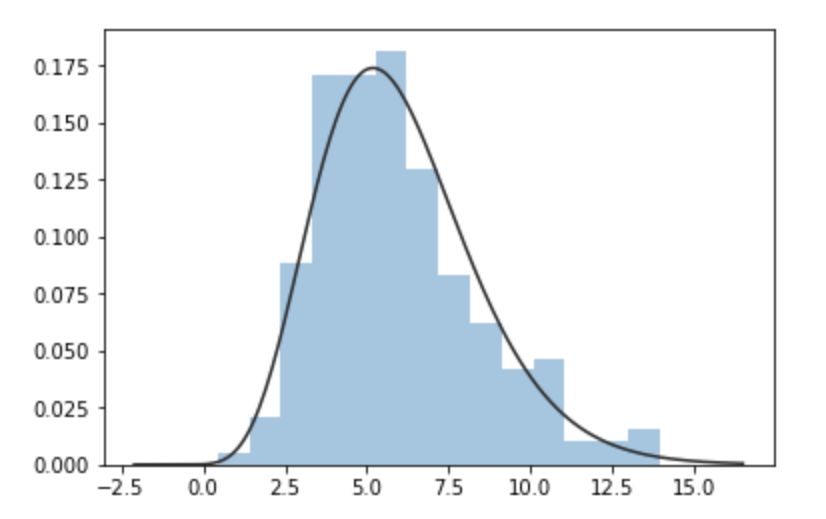## 散点图 jointplot

``````mean, cov = [0, 1], [(1, .5), (.5, 1)]
data = np.random.multivariate_normal(mean, cov, 200)
df = pd.DataFrame(data, columns=['x', 'y'])
df
``````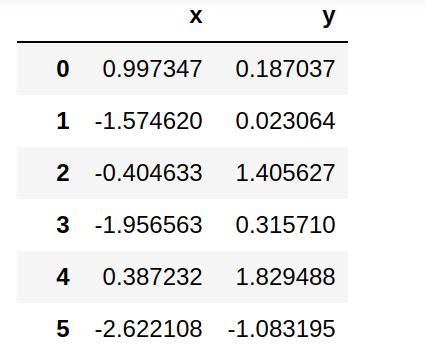``````sns.jointplot(x='x', y='y', data=df)
``````
• x：x 轴所需展示的数据，传入字符串的话表示数据标签，还需传入 data 集
• y：y 轴所需展示的数据，传入字符串的话表示数据标签，还需传入 data 集
• data：数据集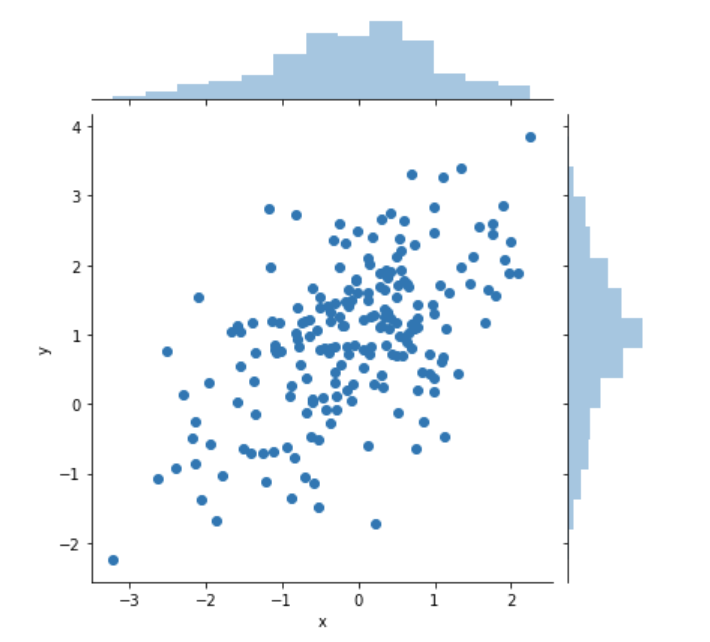``````# 创建1000个数据
x, y = np.random.multivariate_normal(mean, cov, 1000).T

sns.jointplot(x=x, y=y, kind='hex')
``````
• x：x 轴所需展示的数据
• y：y 轴所需展示的数据
•  kind：散点图格式，kind : { “scatter” “reg” “resid” “kde” “hex” }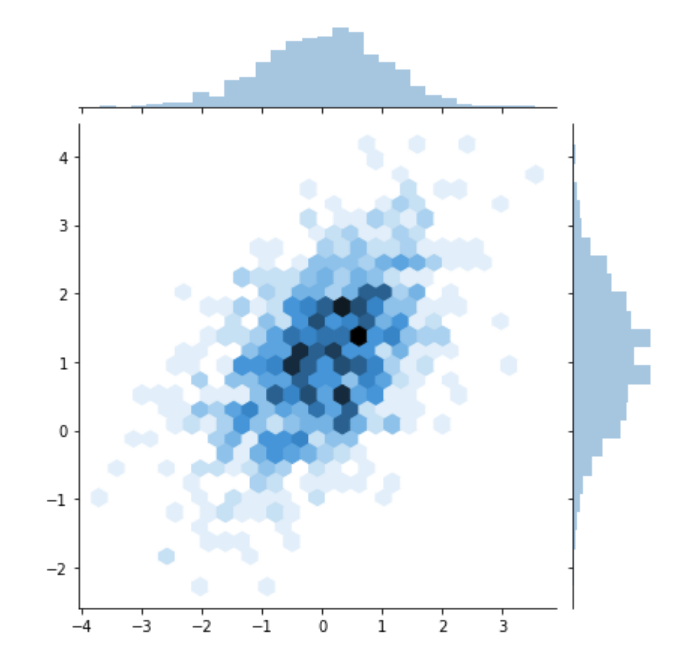### 回归图

• regplot
• lmplot

regplot 是基本的回归图绘制方法，而 lmplot 则提供了一些更高级操作。在分析数据时可以根据不同的需要来使用。

``````tips = sns.load_dataset('tips')
``````

``````tips.head()
``````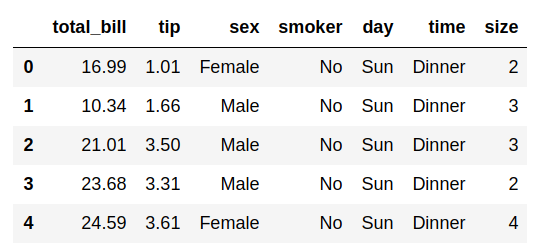• total_bill：总花费金额
• tip：小费金额
• sex：性别
• smoker：是否吸烟
• day：星期日期
• time：时间，中午还是晚上
• size：吃饭人数

#### regplot

``````sns.regplot(x='total_bill', y='tip', data=tips)
``````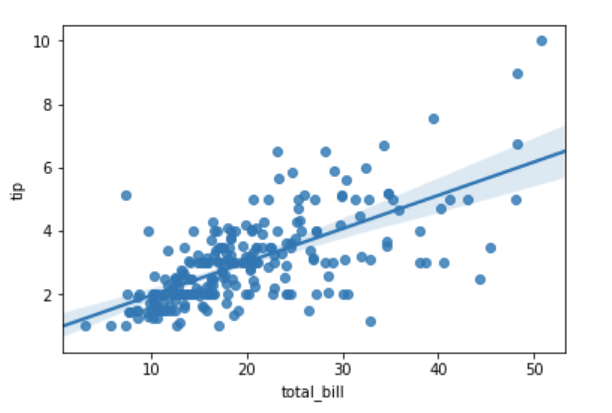``````sns.regplot(x='size', y='tip', data=tips)
````````````sns.regplot(x='size', y='tip', data=tips, x_jitter=0.05)
``````
• x_jitter：左右抖动 0.05 的距离#### lmplot

lmplot 也可以用来绘制回归分析的曲线，而且还拥有较多的可选参数，可以用来绘制出更复杂的图像

#### hue

hue 属性是关注数据集中的变量

``````sns.lmplot(x='total_bill', y='tip', hue='smoker', data=tips)
``````• markers：点的样式
• palette：调色板名称
``````sns.lmplot(x="total_bill", y="tip", hue="smoker", data=tips,
markers=["o", "x"], palette="Set1");
``````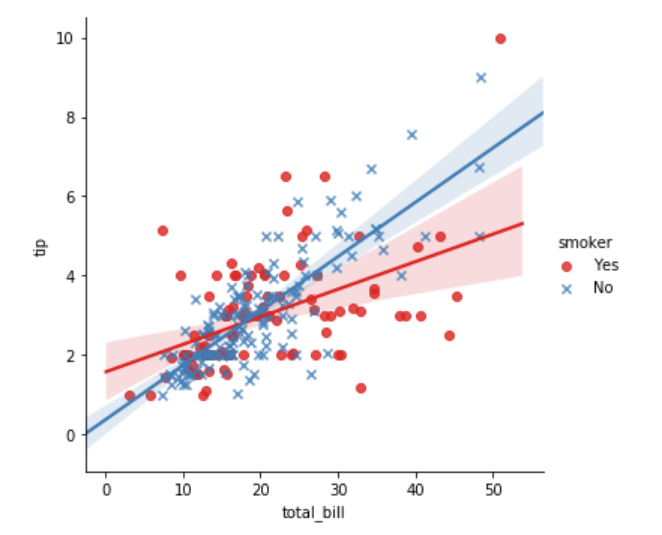#### col

``````sns.lmplot(x='total_bill', y='tip', hue='smoker', col='time', data=tips)
``````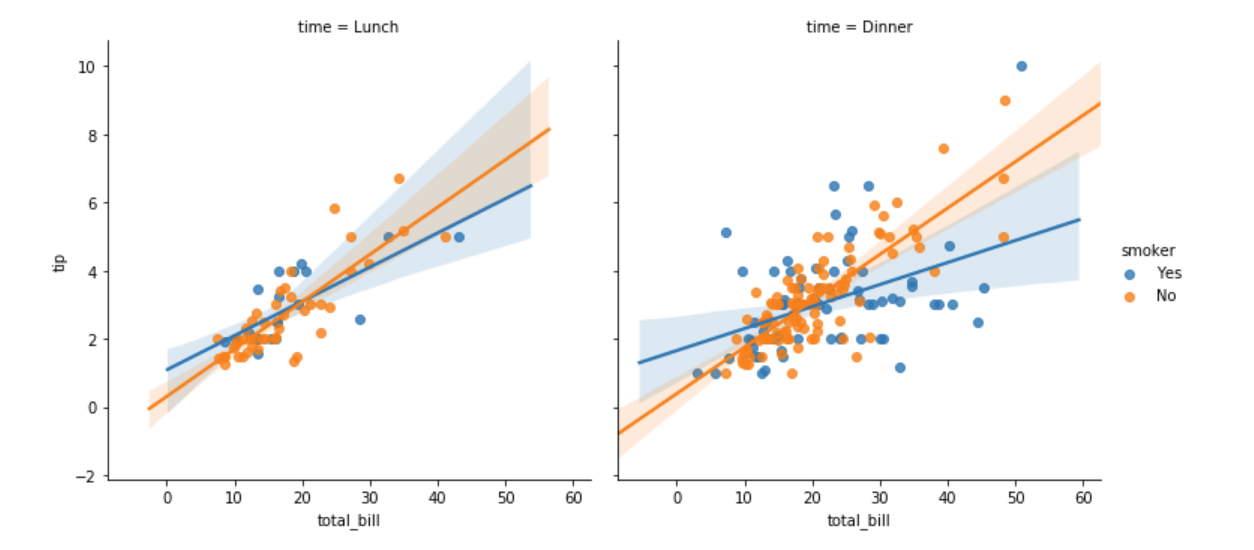#### row

``````sns.lmplot(x='total_bill', y='tip', hue='smoker', col='time', row='sex', data=tips)
``````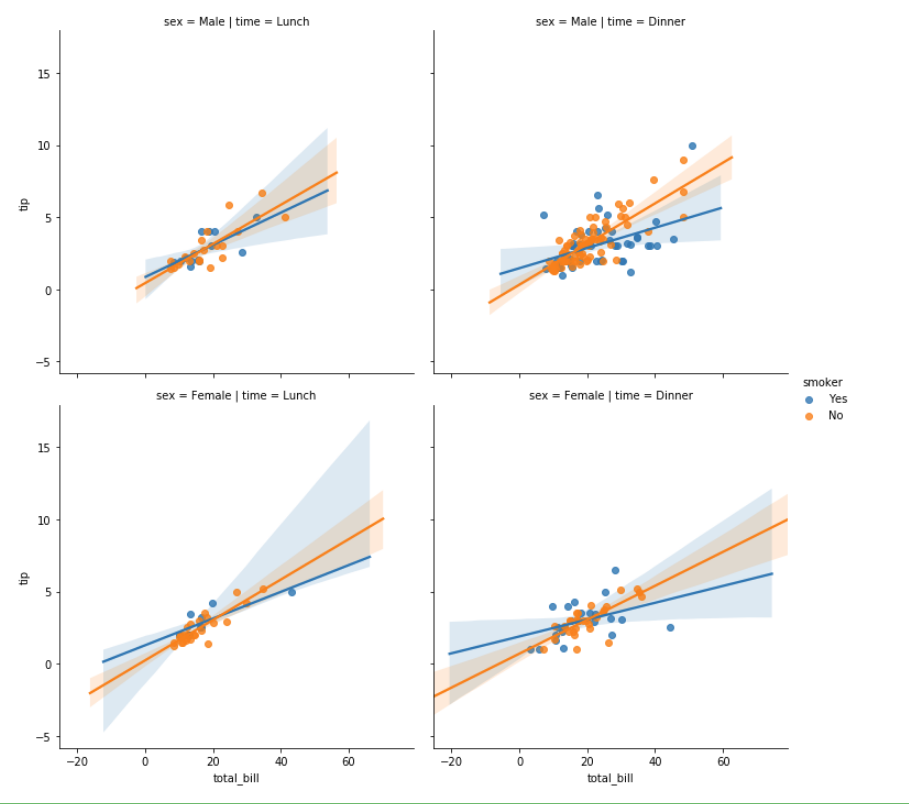• col_wrap：一行展示几个图形，
• size：图形的大小
``````sns.lmplot(x="total_bill", y="tip", col="day", data=tips,
col_wrap=2, size=4);
``````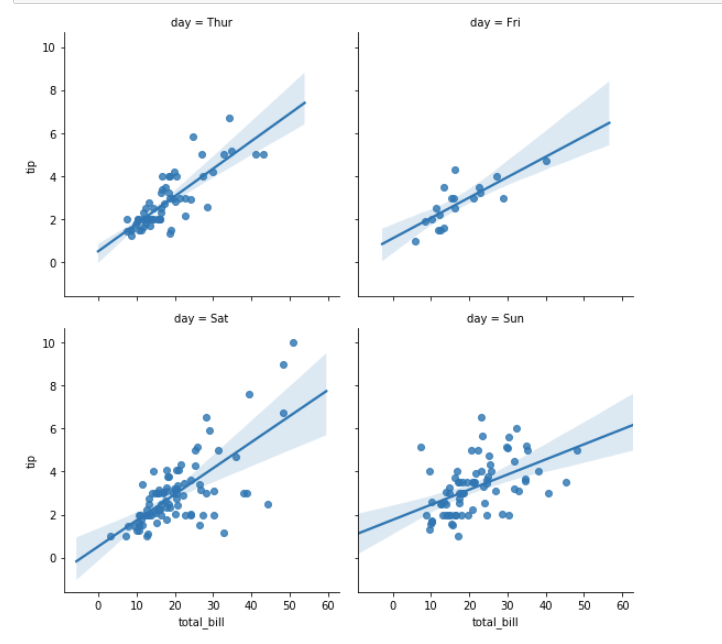## 多变量分析

### 多变量图 pairplot

``````# 加载鸢尾花数据集
iris = sns.load_dataset("iris")
# pairplot 绘制数据集中的多个属性之间的关系。
sns.pairplot(iris)
``````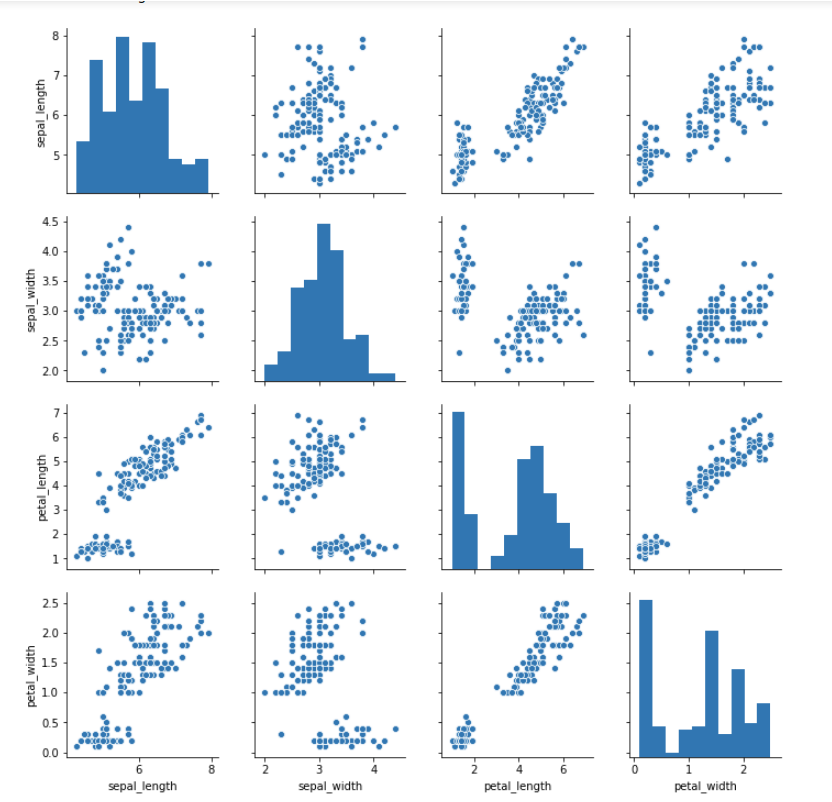## 分类数据

• 分布散点图：stripplot、swarmplot
• 盒图：boxplot
• 小提琴图：violinplot
• 条形图：barplot
• 点图：pointplot
• 多层面板分类图：factorplot

### 分布散点图绘制

``````sns.stripplot(x='day', y='total_bill', data=tips)
``````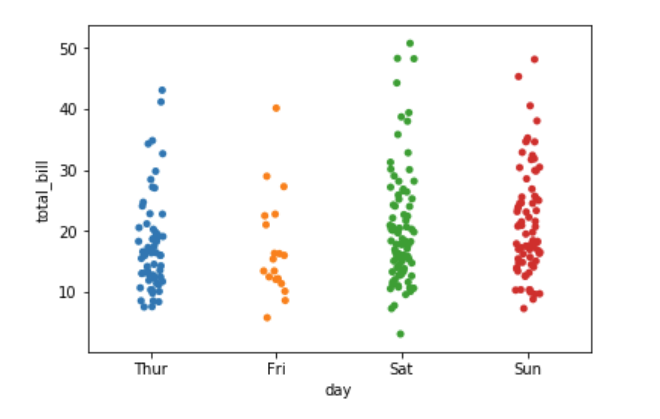``````sns.stripplot(x='day', y='total_bill', data=tips, jitter=False)
``````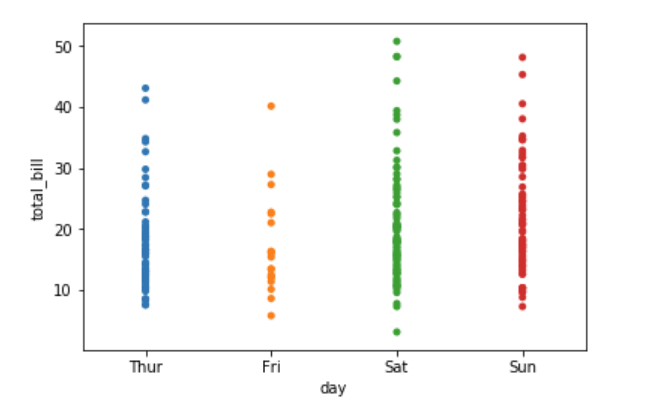``````sns.swarmplot(x='day', y='total_bill', data=tips)
``````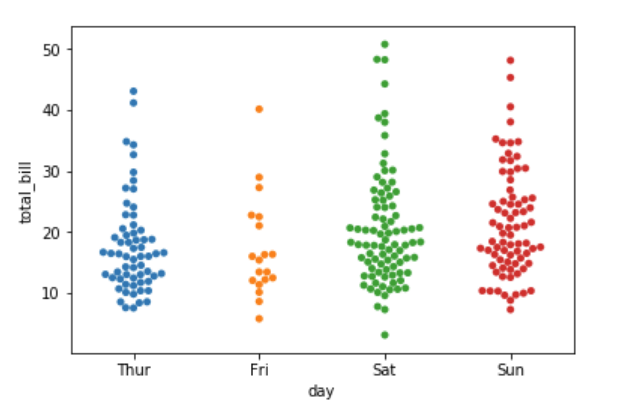``````sns.swarmplot(x='day', y='total_bill', hue='sex', data=tips)
``````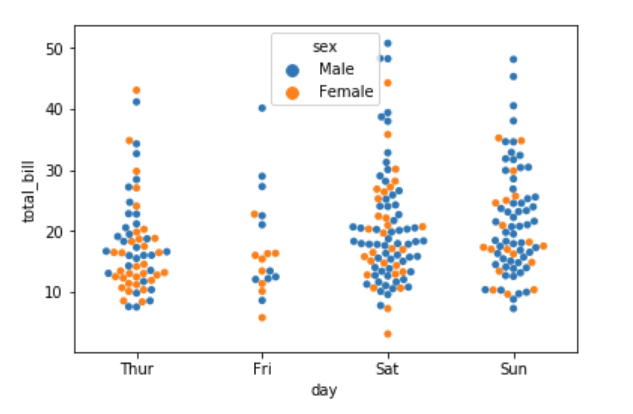``````sns.swarmplot(y='day', x='total_bill', hue='sex', data=tips)
``````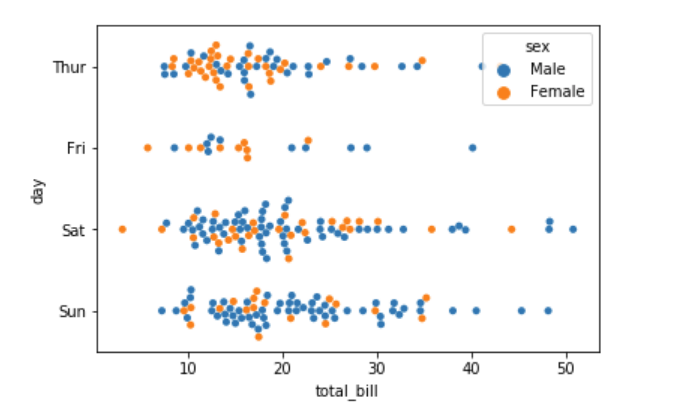### 盒图

• IQR 即统计学概念四分位距，第 1/4 位与第 3/4 位之间的距离
• N = 1.5IQR
• 如果一个值 >Q3+N 或 <Ｑ1-N,则为离群点
``````sns.boxplot(x='day', y='total_bill', hue='time', data=tips)
``````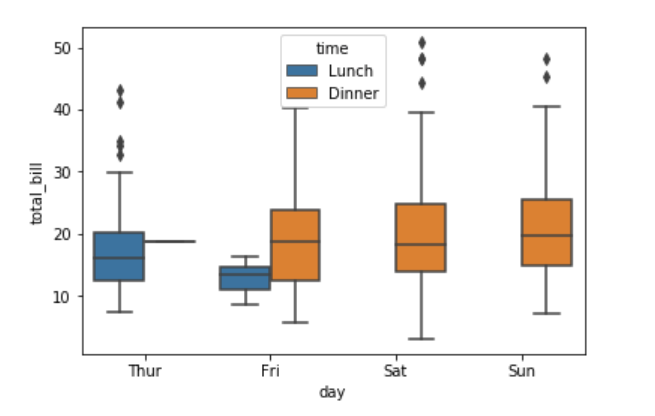``````sns.boxplot(data=iris,orient="h")
``````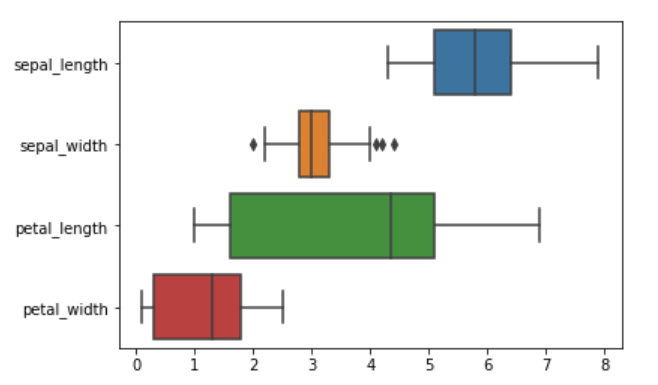### 小提琴图

• 使用核密度估计来更好地描述值的分布。
• 小提琴内还显示了箱体四分位数和晶须值。
• 由于小提琴使用KDE，还有一些其他可以调整的参数，相对于简单的boxplot增加了一些复杂性
``````sns.violinplot(x='day', y='total_bill', hue='time', data=tips)
``````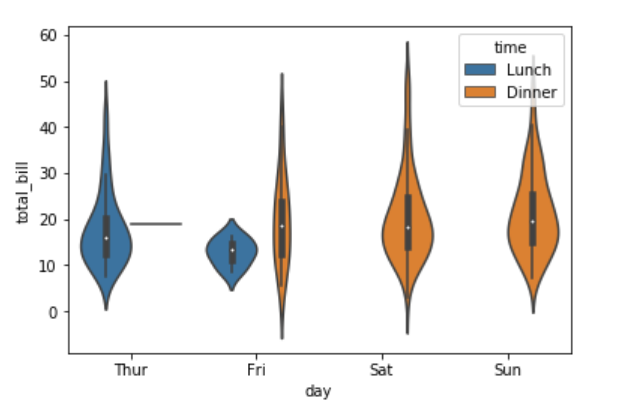``````sns.violinplot(x='day', y='total_bill', hue='sex', data=tips, split=True)
``````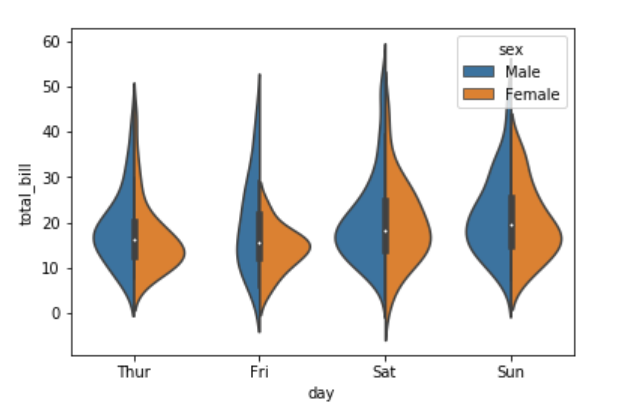### 条形图

• 显示值的集中趋势可以用条形图
``````# 加载所需的数据集
titanic = sns.load_dataset("titanic")

sns.barplot(x="sex", y="survived", hue="class", data=titanic);
``````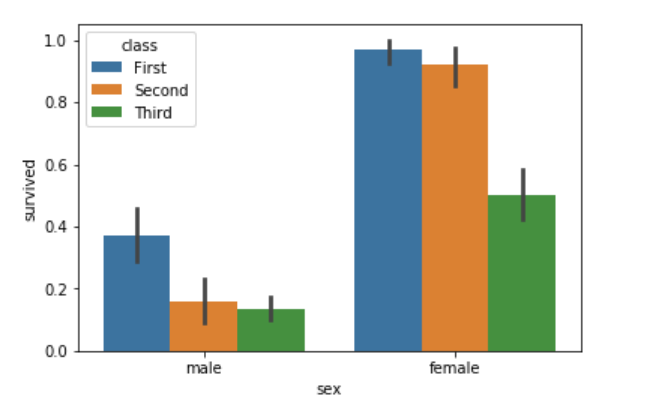### 点图

• 点图可以更好的显示数据的变化
``````sns.pointplot(x="sex", y="survived", hue="class", data=titanic);
``````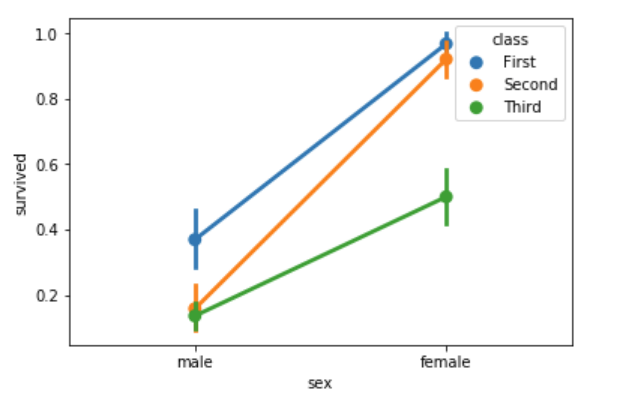``````sns.pointplot(x="class", y="survived", hue="sex", data=titanic,
palette={"male": "g", "female": "m"},
markers=["^", "o"],
linestyles=["-", "--"]);
``````
• palette：调色板
• markers：点的样式
• linestyles：曲线样式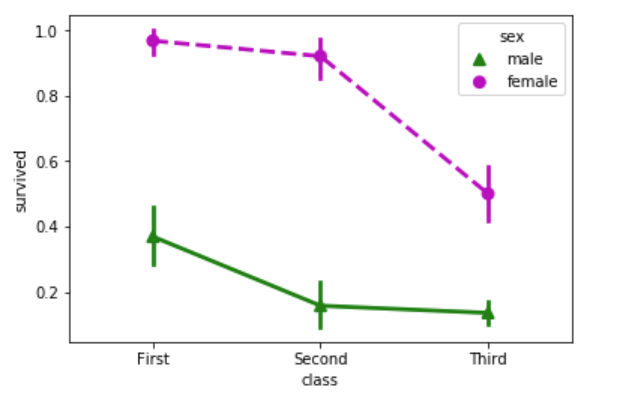### 多层面板分类图

``````seaborn.factorplot(x=None, y=None, hue=None, data=None, row=None, col=None, col_wrap=None, estimator=, ci=95, n_boot=1000, units=None, order=None, hue_order=None, row_order=None, col_order=None, kind='point', size=4, aspect=1, orient=None, color=None, palette=None, legend=True, legend_out=True, sharex=True, sharey=True, margin_titles=False, facet_kws=None, **kwargs)
``````

### Parameters：

• x,y,hue 数据集变量 变量名
• date 数据集 数据集名
• row,col 更多分类变量进行平铺显示 变量名
• col_wrap 每行的最高平铺数 整数
• estimator 在每个分类中进行矢量到标量的映射 矢量
• ci 置信区间 浮点数或None
• n_boot 计算置信区间时使用的引导迭代次数 整数
• units 采样单元的标识符，用于执行多级引导和重复测量设计 数据变量或向量数据
• order, hue_order 对应排序列表 字符串列表
• row_order, col_order 对应排序列表 字符串列表
• kind : 可选：point 默认, bar 柱形图, count 频次, box 箱体, violin 提琴, strip 散点，swarm 分散点 size 每个面的高度（英寸） 标量 aspect 纵横比 标量 orient 方向 “v”/”h” color 颜色 matplotlib颜色 palette 调色板 seaborn颜色色板或字典 legend hue的信息面板 True/False legend_out 是否扩展图形，并将信息框绘制在中心右边 True/False share{x,y} 共享轴线 True/False

``````sns.factorplot(x="day", y="total_bill", hue="smoker", data=tips)
``````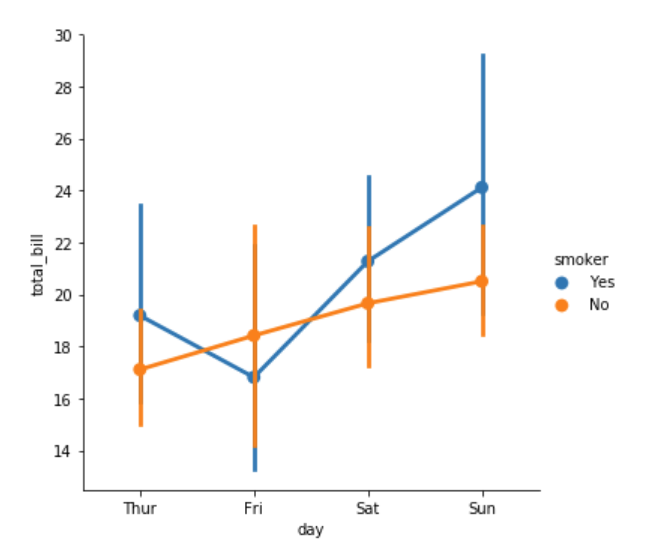``````sns.factorplot(x="day", y="total_bill", hue="smoker", data=tips, kind="bar")
``````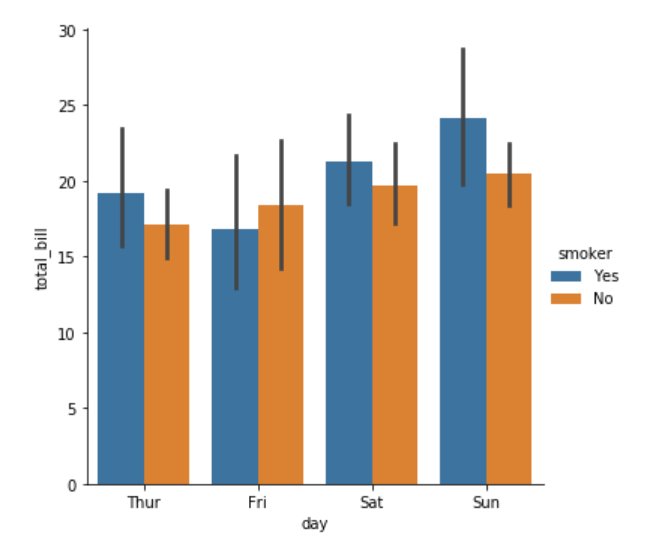``````sns.factorplot(x="day", y="total_bill", hue="smoker",
col="time", data=tips, kind="swarm")
``````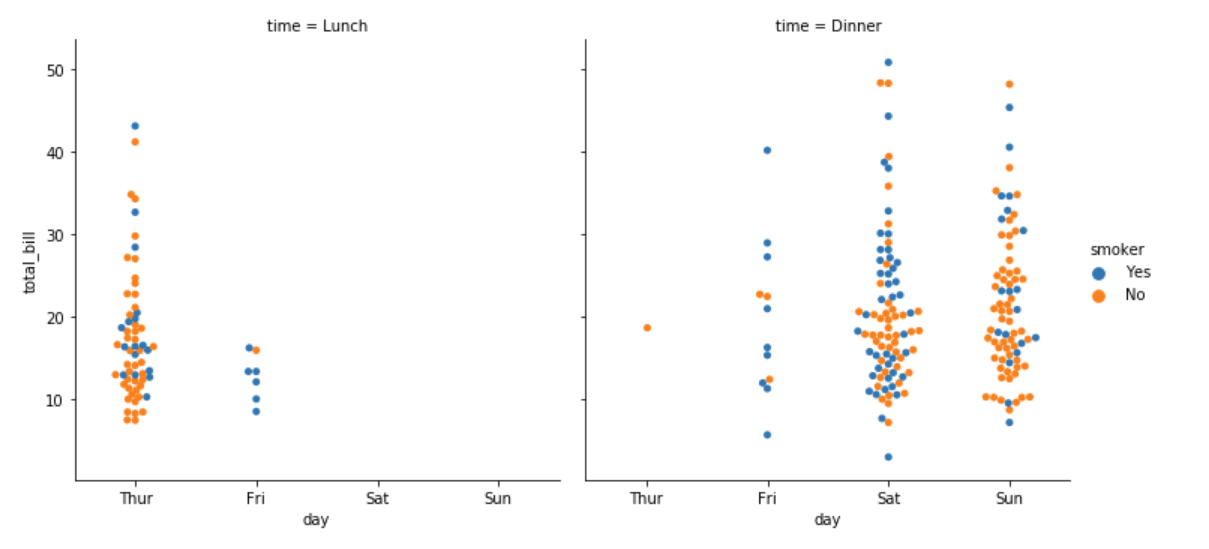``````sns.factorplot(x="time", y="total_bill", hue="smoker",
col="day", data=tips, kind="box")
``````